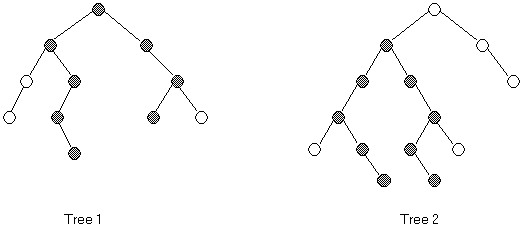Computer Science - C++ Lesson 39:  Binary Tree Practice

OBJECTIVES:

• The student will write recursive algorithms for preorder, inorder, and postorder output.

• The student will write a function to count the nodes in a binary tree

• The student will write a function to count the leaves in a binary tree.

• The student will write a function to determine the height of a binary tree.

• The student will write a function to determine the width of a binary tree.

•   The student will write a function to clear a binary tree, disposing of all memory locations.

The data stored in these binary trees will be single-alphabet characters, loaded from one line text files.  Here are the suggested files.

(FileA.txt) = KECAJGHOSRV

(FileB.txt) = QJHBADFKNLMPTW

Each of these files will produce the binary trees drawn at the top of the lab exercise sheet.  The (FileA.txt) will generate the left picture while (FileB.txt) will generate the right picture.  This will make it easier  to verify if their algorithms are correct.

Lab exercise Treestats

Background:The height of a binary tree is defined as the number of nodes in the longest path from the root to a leaf of the tree.  The heights of the trees above are 5 and 6 for Tree 1 and Tree 2, respectively.

The width of a binary tree is the number of nodes in the longest path in the tree.  The width of an empty tree is 0; the width of a single-node tree is 1; and the width of Tree 1 is 8 (darkened nodes) and the width of Tree 2 is 9.  The longest path may or may not include the root node.  In general, the width of an empty tree is 0 and the width of a nonempty tree is the maximum of the following three quantities:

1.   The width of the left subtree

2.   The width of the right subtree

3.   The length of the longest path that includes the root (which can be calculated from the heights of the left and right subtrees).

When writing these two functions (height and width), you may find it useful to have an auxiliary function ready to use.

int  max (int a, int b)

{

if (a > b)  return a;

else  return b;

}

Assignment:

1.   Write a main menu module with the following menu choices:

(1)     Fill the tree from a file

(2)     Preorder output

(3)     Inorder output

(4)     Postorder output

(5)     Count the nodes in the tree

(6)     Count the leaves in the tree

(7)     Find the height of the tree

(8)     Find the width of the tree

(9)     Clear the tree

(q)     Quit

2.   Your task is to systematically code and test each of these functions.  The data stored in this tree will be a single letter.  The source of the letters will be two different files, each consisting of one line of capital letters:  (fileA.txt) and (fileB.txt).

3.   Clearing the tree must also dispose of the nodes as the tree is cleared.

4.   You should also print appropriate messages, labeling each output.

1.   Prepare these two text files:

(fileA.txt) = KECAJGHOSRV

(fileB.txt) = QJHBADFKNLMPTW

2.   The two files above will generate binary trees having the distinctive shape of the front side of this lab.  This will allow you to test your program and verify the correctness of your answers.